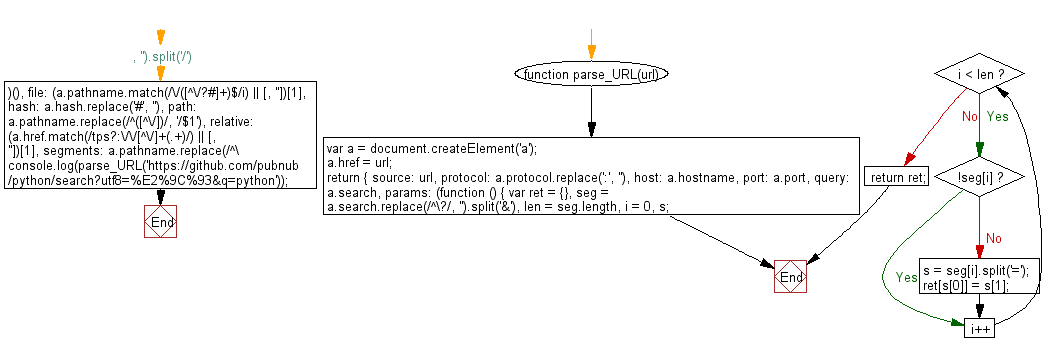# JavaScript: Parse an URL

## JavaScript Object: Exercise-12 with Solution

Write a JavaScript function to parse an URL.

Sample Solution: -

HTML Code:

``````<!DOCTYPE html>
<html>
<meta charset="utf-8">
<title>JavaScript function to parse an URL.</title>
<body>

</body>
</html>
```
```

JavaScript Code:

``````function parse_URL(url) {
var a = document.createElement('a');
a.href = url;
return {
source: url,
protocol: a.protocol.replace(':', ''),
host: a.hostname,
port: a.port,
query: a.search,
params: (function () {
var ret = {},
seg = a.search.replace(/^\?/, '').split('&'),
len = seg.length,
i = 0,
s;
for (; i < len; i++) {
if (!seg[i]) {
continue;
}
s = seg[i].split('=');
ret[s] = s;
}
return ret;
})(),
file: (a.pathname.match(/\/([^\/?#]+)\$/i) || [, '']),
hash: a.hash.replace('#', ''),
path: a.pathname.replace(/^([^\/])/, '/\$1'),
relative: (a.href.match(/tps?:\/\/[^\/]+(.+)/) || [, '']),
segments: a.pathname.replace(/^\//, '').split('/')
};
}

console.log(parse_URL('https://github.com/pubnub/python/search?utf8=%E2%9C%93&q=python'));
```
```

Sample Output:

```{"source":"https://github.com/pubnub/python/search?utf8=%E2%9C%93&q=python","protocol":"https","host":"github.com","port":"","query":"?utf8=%E2%9C%93&q=python","params":{"utf8":"%E2%9C%93","q":"python"},"file":"search","hash":"","path":"/pubnub/python/search","relative":"/pubnub/python/search?utf8=%E2%9C%93&q=python","segments":["pubnub","python","search"]}
```

Flowchart:Live Demo:

See the Pen javascript-object-exercise-12 by w3resource (@w3resource) on CodePen.

Improve this sample solution and post your code through Disqus

What is the difficulty level of this exercise?

﻿

## JavaScript: Tips of the Day

JavaScript: The unary operator

```let num = 10;

const increaseNumber = () => num++;
const increasePassedNumber = number => number++;

const num1 = increaseNumber();
const num2 = increasePassedNumber(num1);

console.log(num1);
console.log(num2);
```

The unary operator ++ first returns the value of the operand, then increments the value of the operand. The value of num1 is 10, since the increaseNumber function first returns the value of num, which is 10, and only increments the value of num afterwards.
num2 is 10, since we passed num1 to the increasePassedNumber. number is equal to 10(the value of num1. Again, the unary operator ++ first returns the value of the operand, then increments the value of the operand. The value of number is 10, so num2 is equal to 10.

Ref: https://bit.ly/323Y0P6